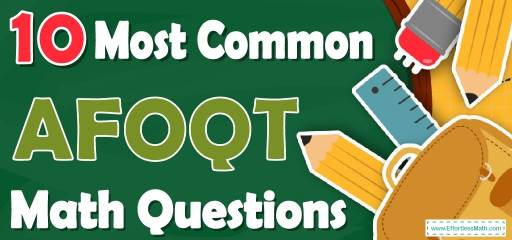# 10 Most Common AFOQT Math QuestionsPreparing for the AFOQT Math test? Want a preview of the most common mathematics questions on the AFOQT Math test? If so, then you are in the right place.

The mathematics section of AFOQT can be a challenging area for many test-takers, but with enough patience, it can be easy and even enjoyable!

Preparing for the AFOQT Math test can be a nerve-wracking experience. Learning more about what you’re going to see when you take the AFOQT can help to reduce those pre-test jitters. Here’s your chance to review the 10 most common AFOQT Math questions to help you know what to expect and what to practice most. Try these 10 most common AFOQT Math questions to hone your mathematical skills and to see if your math skills are up to date on what’s being asked on the exam or if you still need more practice.

Make sure to follow some of the related links at the bottom of this post to get a better idea of what kind of mathematics questions you need to practice.

## 10 Sample AFOQT Math Practice Questions

1- In the deck of cards, there are 4 spades, 3 hearts, 7 clubs, and 10 diamonds. What is the probability that William will pick out a spade?

A. $$\frac{1}{6}$$

B. $$\frac{1}{8}$$

C. $$\frac{1}{9}$$

D. $$\frac{1}{5}$$

2- A writer finishes 180 pages of his manuscript in 20 hours. How many pages is his average per hour?

A. 18

B. 14

C. 12

D. 9

3- What is the volume of a box with the following dimensions?
Height = 4 cm Width = 5 cm Length = 6 cm

A. 15 cm$$^3$$

B. 60 cm$$^3$$

C. 90 cm$$^3$$

D. 120 cm$$^3$$

4- Simplify $$6x^2 y^3 (2x^2 y)^3=$$?

A. $$12x^4 y^6$$

B. $$12x^8 y^6$$

C. $$48x^4 y^6$$

D. $$48x^8 y^6$$

5- A writer finishes 240 pages of his manuscript in 60 hours. How many pages is his average per hour?

A. 15

B. 12

C. 6

D. 4

6- Ava needs $$\frac{1}{5}$$ of an ounce of salt to make 1 cup of dip for fries. How many cups of dip will she be able to make if she has 50 ounces of salt?

A. 35

B. 200

C. 75

D. 250

7- What is the median of these numbers? 2, 27, 28, 19, 67, 44, 35

A. 19

B. 28

C. 35

D. 44

8- If $$a = 3$$, what is the value of b in this equation?

A. 10

B. 8

C. 6

D. 4

9- What is the value of $$x$$ in the following equation?
$$\frac{2}{3} x+\frac{1}{6}= \frac{1}{3}$$

A. 6

B. $$\frac{1}{2}$$

C. $$\frac{1}{3}$$

D. $$\frac{1}{4}$$

10- In the following right triangle, what is the value of $$x$$ rounded to the nearest hundredth?

A. 23.24

B. 2.33

C. 10.29

D. 6.40

## Best AFOQT Math Prep Resource for 2022

1- A
probability $$=\frac{desired \space outcomes}{possible \space outcomes}=\frac{4}{4+3+7+10}=\frac{4}{24}=\frac{1}{6}$$

2- D
$$\frac{180}{20}=9$$

3- D
Volume of a box = length $$\times$$ width $$\times$$ height = 4 $$\times$$ 5 $$\times$$ 6 = 120

4- D
Simplify.
$$6x^2 y^3 (2x^2 y)^3= 6x^2 y^3 (8x^6 y^3 ) = 48x^8 y^6$$

5- D
average $$=\frac{sum}{total}=\frac{240}{60}=4$$

6- D
Write a proportion and solve.
$$\frac{\frac{1}{5}}{1}=\frac{50}{x}$$
$$x=\frac{50}{\frac{1}{5}}= 250$$

7- B
Write the numbers in order:
2, 19, 27, 28, 35, 44, 67
Median is the number in the middle. So, the median is 28

8- C
If $$a = 3$$ then:
$$b = \frac{a^2}{3}+ 3 ⇒ b =\frac{3^2}{3}+ 3 = 3 + 3 = 6$$

9- D
Isolate and solve for $$x$$.
$$\frac{2}{3} x+\frac{1}{6} = \frac{1}{3} {\Rightarrow} \frac{2}{3} x= \frac{1}{3} -\frac{1}{6} = \frac{1}{6} {\Rightarrow} \frac{2}{3} x= \frac{1}{6}$$
Multiply both sides by the reciprocal of the coefficient of $$x$$.
$$(\frac{3}{2}) \frac{2}{3} x= \frac{1}{6} (\frac{3}{2}) {\Rightarrow} x= \frac{3}{12}=\frac{1}{4}$$

10- D
Use Pythagorean Theorem: $$a^2 + b^2 = c^2$$
$$(4)^2 + (5)^2 = c^2 ⇒ 16 + 25 = 41 = C^2$$
$$C = \sqrt{41} = 6.403$$

Looking for the best resource to help you succeed on the AFOQT Math test?

## More from Effortless Math for AFOQT Test …

### Do you know what kind of Math is on the AFOQT Test?

We briefly review the contents of these two subtests in the following post: What Kind of Math Is on the AFOQT Test?

### Looking for AFOQT Math websites to find FREE online resources?

Here is our complete list of Top 10 Websites for FREE AFOQT Math Preparation.

Have a look at our Full-Length AFOQT Math Practice Test and Free AFOQT Math Practice Test.

## Have any questions about the AFOQT Test?

### What people say about "10 Most Common AFOQT Math Questions - Effortless Math: We Help Students Learn to LOVE Mathematics"?

No one replied yet.

X
49% OFF

Limited time only!

Save Over 49%

SAVE $34 It was$69.99 now it is \$35.99